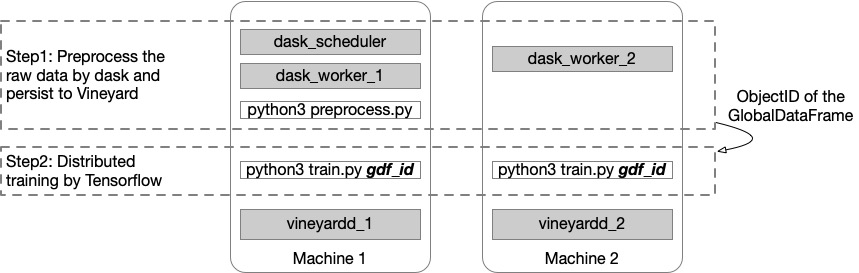The integration with Dask enables dask.array and dask.dataframe to be seamlessly persisted in and retrieved from Vineyard. In the following sections, we demonstrate how Vineyard simplifies the implementation of an example that utilizes Dask for data preprocessing and TensorFlow for distributed learning, as previously showcased in the blog.

## The Deployment#As illustrated in the figure above, we employ two machines for the distributed tasks for demonstration purposes. The Vineyard daemon processes are launched on both machines, along with the Dask workers. The Dask scheduler is initiated on the first machine, where we also run the Dask preprocessing program in the first step, as the Dask scheduler manages the distribution of computation tasks among its workers.

In the second step, we execute the training program on both machines with different TF_CONFIG settings. For details on configuring the setup, please refer to the documentation.

In this step, we load the mnist data and duplicate it to simulate the parallel processing as same as the blog.

```from vineyard.core.builder import builder_context

def get_mnist():
# The `x` arrays are in uint8 and have values in the [0, 255] range.
# You need to convert them to float64 with values in the [0, 1] range.
x_train = x_train / np.float64(255)
y_train = y_train.astype(np.int64)
return pd.DataFrame({'x': list(x_train), 'y': y_train})

datasets = [delayed(get_mnist)() for i in range(20)]
dfs = [dd.from_delayed(ds) for ds in datasets]
gdf = dd.concat(dfs)

return gdf_id
```

Here the returned gdf_id is the ObjectID of a vineyard::GlobalDataFrame which consists of 20 partitions (10 partitions on each machine).

## Training in Tensorflow#

In this step, we use the preprocessed data gdf_id to train a model distributedly in keras of Tensorflow.

```from vineyard.contrib.ml.tensorflow import register_tf_types
from vineyard.core.resolver import resolver_context

def mnist_dataset(gdf_id, batch_size):
with resolver_context() as resolver:
# register the resolver for tensorflow Dataset to the resolver_context
register_tf_types(None, resolver)
train_datasets = vineyard.connect().get(gdf_id, data='x', label='y')
train_datasets = train_datasets.repeat().batch(batch_size)

options = tf.data.Options()
options.experimental_distribute.auto_shard_policy = tf.data.experimental.AutoShardPolicy.OFF
train_datasets_no_auto_shard = train_datasets.with_options(options)
return train_datasets_no_auto_shard

def build_and_compile_cnn_model():
model = tf.keras.Sequential([
tf.keras.layers.InputLayer(input_shape=(28, 28)),
tf.keras.layers.Reshape(target_shape=(28, 28, 1)),
tf.keras.layers.Conv2D(32, 3, activation='relu'),
tf.keras.layers.Flatten(),
tf.keras.layers.Dense(128, activation='relu'),
tf.keras.layers.Dense(10)
])
model.compile(
loss=tf.keras.losses.SparseCategoricalCrossentropy(from_logits=True),
optimizer=tf.keras.optimizers.SGD(learning_rate=0.001),
metrics=['accuracy'])
return model

def train(gdf_id):
per_worker_batch_size = 64
strategy = tf.distribute.MultiWorkerMirroredStrategy()
train_dataset = mnist_dataset(gdf_id, per_worker_batch_size)

with strategy.scope():
multi_worker_model = mnist.build_and_compile_cnn_model()

multi_worker_model.fit(train_dataset, epochs=3, steps_per_epoch=70)
```

To utilize the preprocessed data, we first register the resolvers capable of resolving a vineyard::GlobalDataFrame distributed across multiple workers within the resolver_context. Subsequently, we can directly obtain the tf.data.Dataset from Vineyard using the get method.

Note

It is essential to specify the column names for the data and label, as they were set in the previous step.

## Transfer Learning#

In this section, we demonstrate how the dask-vineyard integration can be effectively utilized in transfer learning scenarios. Transfer learning is a technique where a pre-trained deep learning model is used to compute features for downstream models. Storing these features in memory is advantageous, as it eliminates the need to recompute features or incur significant I/O costs by repeatedly reading them from disk. We will refer to the featurization example and use the tf_flowers dataset as a dask.array. We will employ the pre-trained ResNet50 model to generate features and subsequently store them in Vineyard. The resulting global tensor in Vineyard will consist of 8 partitions, each containing 400 data slots.

```def get_images(idx, num):
paths = list(Path("flower_photos").rglob("*.jpg"))[idx::num]
data = []
for p in paths:
with open(p,'rb') as f:
arr = preprocess_input(img_to_array(img))
data.append(arr)
return np.array(data)

def featurize(v, block_id=None):
model = ResNet50(include_top=False)
preds = model.predict(np.stack(v))
return preds.reshape(400, 100352)

imgs = [da.from_delayed(delayed(get_images)(i,8), shape=(400, 244, 244, 3), dtype='float') for i in range(8)]
imgs = da.concatenate(imgs, axis=0)
res = imgs.map_blocks(featurize, chunks=(400,100352), drop_axis=[2,3], dtype=float)